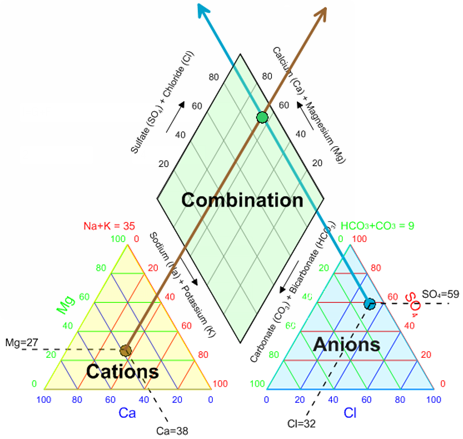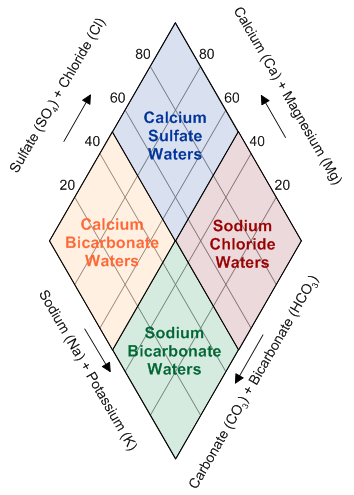Follow

# What is a piper plot (trilinear diagram)?

In the fields of hydrogeology and groundwater analysis, piper plots (also known as trilinear diagrams) are very powerful tools for visualizing the relative abundance of common ions in water samples. Although there are other plot types that can show abundance of ions in groundwater, this plot type is especially useful because it allows you to plot multiple samples on the same plot, thus allowing for grouping water samples by groundwater facies and other criteria. In this day and age, when groundwater is so closely monitored, it is especially important to have a plot type like the piper plot that makes it easy to determine whether it is suitable for human use.

A piper plot is comprised of three components: a ternary diagram in the lower left representing cations (magnesium, calcium, and sodium plus potassium), a ternary diagram in the lower right representing anions (chloride, sulfate, and carbonate plus bicarbonate), and a diamond plot in the middle which is a matrix transformation of the two ternary diagrams. Each sample is normalized to 100 (sum of cations = 100 and sum of anions = 100), so the relative concentrations are on a percentage basis.The three components of the piper plot. Bottom left is a ternary plot of the cations, bottom right is a ternary plot of the anions, and top is a diamond plot of a projection from the other two plots.

The diamond plot then can be analyzed to tell you what kind of groundwater you’re looking at. Samples in the top quadrant are calcium sulfate waters, samples in the left quadrant are calcium bicarbonate waters, samples in the right quadrant are sodium chloride waters, and samples in the bottom quadrant are sodium bicarbonate waters.Interpretation of the diamond plot (modified from http://inside.mines.edu/~epoeter/_GW/18WaterChem2
/WaterChem2pdf.pdf
). Samples in the top quadrant are calcium sulfate waters, which are typical of gypsum ground water and mine drainage. Samples in the left quadrant are calcium bicarbonate waters, which are typical of shallow fresh ground water. Samples in the right quadrant are sodium chloride waters, which are typical of marine and deep  ancient ground water. Samples in the bottom quadrant are sodium bicarbonate waters, which are typical of deep ground water influenced by ion exchange.

For information on creating piper plots, see the Can Grapher create a Piper Plot? If so, how? knowledge base article. For information on creating and editing piper plots, see the Piper Plots (Trilinear Diagram) training video.

Updated September 19, 2018